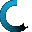Retrieving data ...
Please do not click anywhere else.

If you see no response after 2-3 minutes, then click on the [X] button in the upper right corner to close the window and retry the operation at a later time.
Interactive Multimedia Learning & Teaching Management System Suite## Course Contents

Course Name: Elementary and Middle School Division - Online
• Elementary and Middle School Division
•  1.1 Introduction to Division
•  1.2 Division in Relation to Subtraction
•  1.3 Division in Relation to Multiplication
•  1.4 Division Using Objects
•  1.5 Division with a Remainder - Part 1
•  1.6 Division with 0 and 1
•  1.7 Dividing Using the Division Bracket
•  1.8 Division with a Remainder - Part 2
•  1.9 Division of a 1-digit Number by another 1-digit Number
•  1.10 Division of a 2-digit Number by a 1-digit Number
•  1.11 Division of a 2-digit Number by another 2-digit Number
•  1.12 Division of a 3-digit Number by a 1-digit Number
•  1.13 Division of a 3-digit Number by a 2-digit Number
•  1.14 Dividing a 4-digit Number by a 1-digit Number
•  1.15 Dividing a 4-digit Number by a 2-digit Number